|     |   0 浏览

## 7.1 超过光速的移动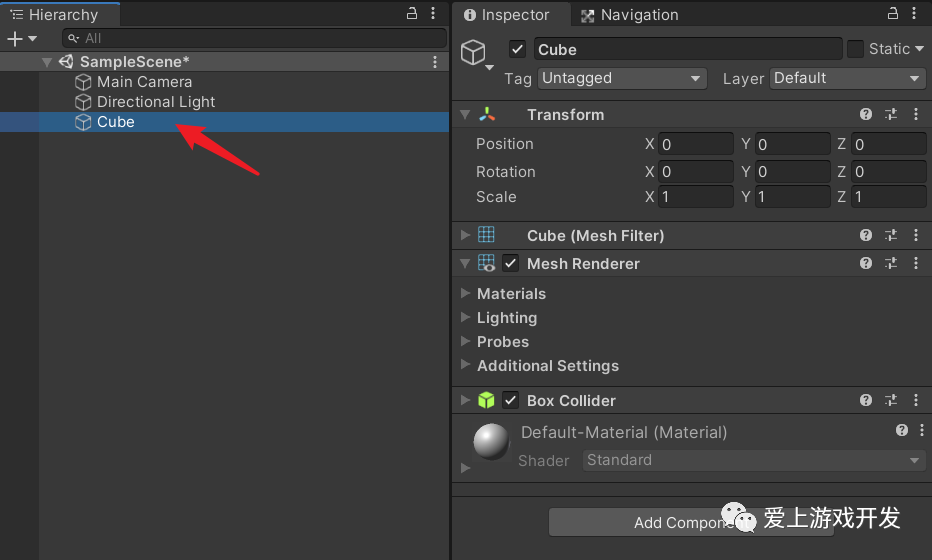接着，创建一个脚本`TransformTest.cs`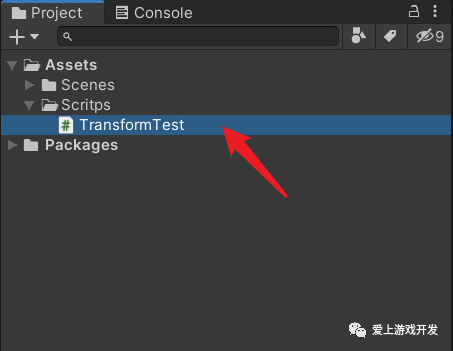``````using System.Diagnostics;
using UnityEngine;

public class TransformTest : MonoBehaviour
{
void Update()
{

if (Input.GetKeyDown(KeyCode.Space))
{

Stopwatch sw = new Stopwatch();

sw.Start();

SetPos();

sw.Stop();

UnityEngine.Debug.Log("总耗时: " + sw.ElapsedMilliseconds);
}
}

void SetPos()
{

Transform selfTransform = transform;

for (int i = 0; i < 100000; ++i)
{
selfTransform.position = Vector3.one * i;
}
}
}
``````

`TransformTest`脚本挂到`Cube`上。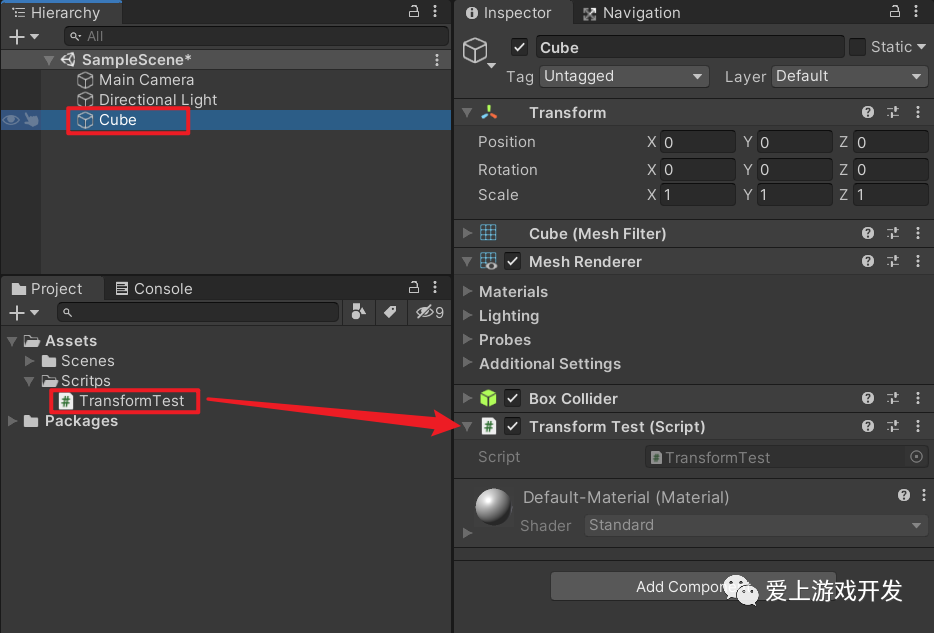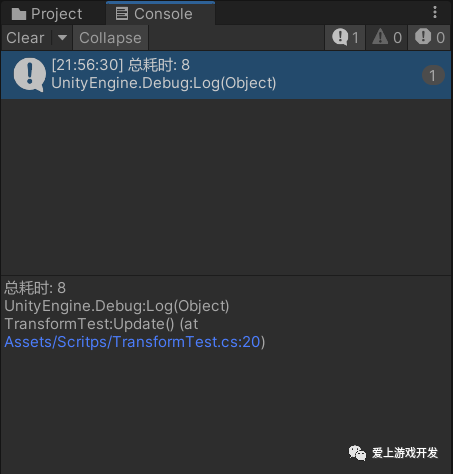``````transform.position = Vector3.zero;

transform.position = new Vector3(241, 0, 0);
``````

## 7.2 初识Transform类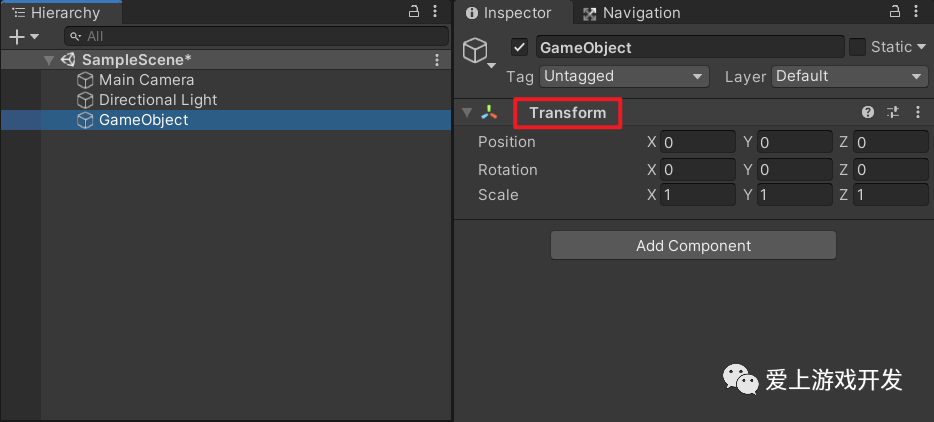## 7.3 Transform的属性

| 属性 | 数据类型 | 描述 |
| - | - | - |
| position | Vector3 | 在世界空间中的坐标 |
| localPosition | Vector3 | 相对于父节点的局部坐标，如果没有父节点，则localPosition等于position |
| eulerAngles | Vector3 | 世界坐标系中的旋转（欧拉角） |
| localEulerAngles | Vector3 | 相对于父节点的局部旋转（欧拉角），如果没有父节点，则localEulerAngles等于eulerAngles |
| rotation | Quaternion | 世界坐标系中的旋转（四元数） |
| localRotation | Quaternion | 相对于父节点的旋转（四元数），如果没有父节点，则localRotation等于rotation |
| right | Vector3 | 局部坐标系的x轴方向向量 |
| up | Vector3 | 局部坐标系的y轴方向向量 |
| forward | Vector3 | 局部坐标系的z轴方向向量 |
| localScale | Vector3 | 相对于父节点的缩放比例 |
| parent | Transform | 父节点的Transform组件 |
| root | Transform | 根节点的Transform组件 |
| childCount | int | 子节点数量 |
| lossyScale | Vector3 | 全局缩放比例（只读） |
| worldToLocalMatrix | Matrix4x4 | 矩阵变换的点从世界坐标转为自身坐标（只读） |
| localToWorldMatrix | Matrix4x4 | 矩阵变换的点从自身坐标转为世界坐标（只读） |

### 7.3.1 设置坐标

``````transform.position = new Vector3(100, 0, 0);
``````

``````transform.localPosition = new Vector3(100, 0, 0);12
``````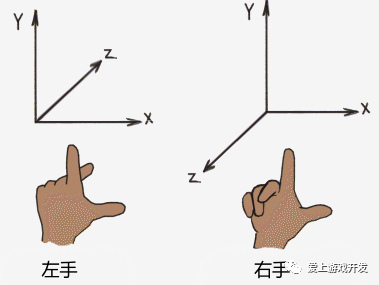### 7.3.2 设置旋转角度

``````transform.localEulerAngles = new Vector3(100, 0, 0);
``````

``````transform.localRotation = Quaternion.Euler(new Vector3(100, 0, 0));
``````

### 7.3.3 设置缩放

``````transform.localScale = Vector3.one * 2;
``````

##### 7.3.4 设置朝向

``````transform.right = new Vector3(1, 1, 0);
``````

``````transform.up = Vector3.up;
``````

``````transform.forward = -Camera.main.transform.forward;
``````

### 7.3.5 设置父节点

``````GameObject parentGo = new GameObject("parentGo");

GameObject childGo = new GameObject("childGo");

childGo.transform.parent = parentGo.transform;
``````

## 7.4 Transform的函数

| 函数 | 说明 |
| - | - |
| Translate | 用来移动物体的函数 |
| Rotate | 用来旋转物体的函数 |
| RotateAround | 让物体以某一点为轴心成圆周运动 |
| LookAt | 让物体的z轴看向目标物体 |
| TransformDirection | 从本地坐标到世界坐标变换方向 |
| InverseTransformDirection | 从世界坐标到本地坐标变换方向，与TransformDirection相反 |
| TransformPoint | 将基于当前游戏对象的局部坐标转化为基于世界坐标系的坐标 |
| InverseTransformPoint | 将基于世界坐标系的坐标转换为基于当前对象的局部坐标 |
| DetachChildren | 分离子物体，所有子物体解除父子关系 |
| Find | 通过名字查找子物体并返回它 |
| SetParent | 设置父节点 |
| IsChildOf | 判断自身是否是某个Transform的子节点 |

### 7.4.1 移动物体

``````public void Translate(float x, float y, float z);
public void Translate(float x, float y, float z, [DefaultValue("Space.Self")] Space relativeTo);
public void Translate(Vector3 translation);
public void Translate(Vector3 translation, [DefaultValue("Space.Self")] Space relativeTo);
public void Translate(float x, float y, float z, Transform relativeTo);
public void Translate(Vector3 translation, Transform relativeTo);
``````

``````m_selfTrans.Translate(1, 0, 0, Space.Self);1
``````

``````public enum Space
{
World = 0,
Self = 1
}
``````

``````public void Translate(Vector3 translation, Transform relativeTo);
``````

### 7.4.2 旋转物体

``````public void Rotate(float xAngle, float yAngle, float zAngle);
public void Rotate(Vector3 eulers, [DefaultValue("Space.Self")] Space relativeTo);
public void Rotate(Vector3 eulers);
public void Rotate(float xAngle, float yAngle, float zAngle, [DefaultValue("Space.Self")] Space relativeTo);
public void Rotate(Vector3 axis, float angle, [DefaultValue("Space.Self")] Space relativeTo);
public void Rotate(Vector3 axis, float angle);
``````

``````transform.Rotate(0, 1, 0);
``````

``````public void RotateAround(Vector3 point, Vector3 axis, float angle);
public void RotateAround(Vector3 axis, float angle);
``````

``````using UnityEngine;

public class TransformTest : MonoBehaviour
{
private Transform m_selfTrans;

void Awake()
{

m_selfTrans = transform;
}

void Update()
{

m_selfTrans.RotateAround(Vector3.zero, Vector3.up, 1);
}
}
``````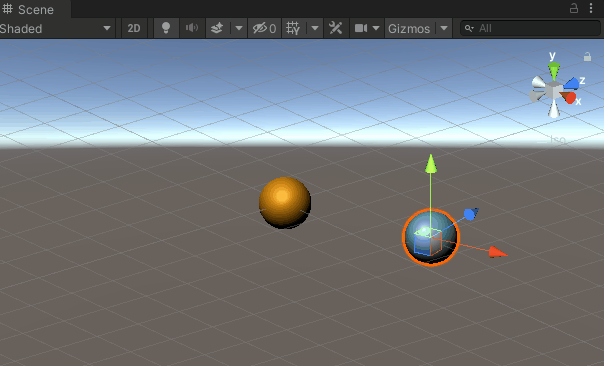### 7.4.3 方向转换计算

``````public Vector3 TransformDirection(float x, float y, float z);
public Vector3 TransformDirection(Vector3 direction);
``````

``````Vector3 worldObjForward = transform.TransformDirection(transform.forward);
``````

``````public Vector3 InverseTransformDirection(Vector3 direction);
public Vector3 InverseTransformDirection(float x, float y, float z);
``````

``````Vector3 localForward = transform.InverseTransformDirection(Vector3.forward);
``````

### 7.4.4 坐标转换计算

``````public Vector3 TransformPoint(float x, float y, float z);
public Vector3 TransformPoint(Vector3 position);
``````

``````Vector3 worldPos = transform.TransformPoint(10, 0, 0);
``````

``````public Vector3 InverseTransformPoint(float x, float y, float z);
public Vector3 InverseTransformPoint(Vector3 position);
``````

``````Vector3 localPos = transform.InverseTransformPoint(10, 0, 0);
``````

### 7.4.5 查找子物体

``````public Transform Find(string n);
``````

`root`节点挂`TransformTest`脚本，脚本代码如下

``````using UnityEngine;

public class TransformTest : MonoBehaviour
{
Transform m_selfTrans;

void Awake()
{

m_selfTrans = transform;
}

void Start()
{

var a = m_selfTrans.Find("a");

var c = m_selfTrans.Find("a/b/c");

var d = m_selfTrans.Find("d");
}
}
``````

### 7.4.6 判断是否是子节点

``````public bool IsChildOf([NotNull] Transform parent);
``````

``````GameObject a = new GameObject("a");
GameObject b = new GameObject("b");
b.transform.parent = a.transform;

if(b.transform.IsChildOf(a.transform))
{
Debug.Log("a 是 b 的子节点");
}
``````

-----------------------------